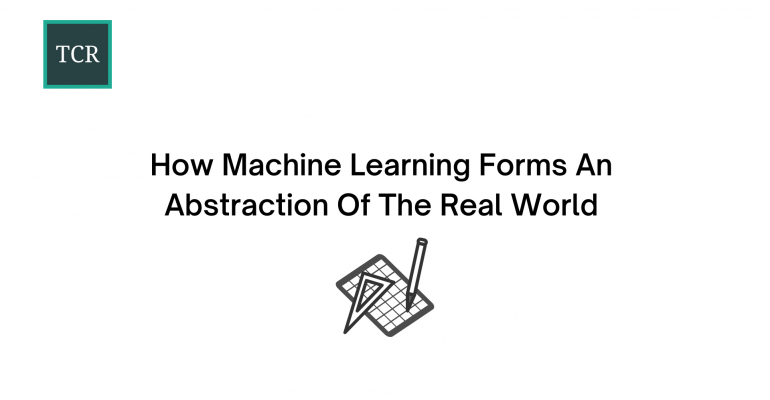Thanks to theidioms.com

## How Machine Learning Forms An Abstraction Of The Real World# How Machine Learning Forms An Abstraction Of The Real WorldIn this article, you will learn how numbers, algebraic notations, visualizations, and Machine Learning all tie together to form an abstraction of the world we live in.

If you’re someone who isn’t great in mathematics or are new to Machine Learning, hopefully, you’ll be charmed by both of them by the time you reach the end of this article.

## What is Abstraction?

We often come across the word ‘abstract’ or ‘abstraction’ when we talk about paintings or simply, works of art. These works of art provide a high-level picture of what a particular thing looks like in the mind of the artist who made the piece of art.

To understand the concept of abstraction in a more general sense, think about a ‘dog’ and try to visualize what comes into your head.

Irrespective of the actual breed, the word ‘dog’ is an abstraction of a furry four-legged animal with a tail that likes belly rubs (usually). So, we use the word ‘dog’ to simply communicate the idea of the animal amongst each other and have already developed a general understanding of what it is.

So, an abstraction is a representative model of any component(s) of the world that we live in. It is used to represent the real-world component through various mediums such as language, art, etc.

## Performing Abstraction using Mathematics

Mathematics uses the concept of abstraction to form a general idea of what different components of the real world look like in terms of numbers, algebraic notations, or graphical visualizations.

Consider a scenario where you go into a shop and find that there’s a 50% discount across all the items in the shop. Excited by the sale, you decide on buying a pair of jeans and you look at the price tag and it says 30 dollars. Now, mentally, you can do the math and find that the discount that you will get is 3 since 50% of 30 dollars = 15 dollars.

Now, let’s say you wanted to buy more than one item in the shop. So, to make this process of calculating the discount amount easier, you can simply form a mathematical equation, such as the following:wherestands for the price of any individual item that you want to buy andstands for the discount amount. This is an example of how mathematics can be used to form an abstraction of the real world.

Continuing on with the example, let’s say we find 5 different items in the store whose prices (in dollars) are 10, 20, 30, 40, and 50.

So, each time we plug the value ofin our equation, we get the values ofas 5, 10, 15, 20, and 25 respectively.

Now, if we plot these values in a graph, it will be clear to you about the effect of the discount percentage on the original amount of the items.

Here, the x-axis represents the original amount of items in dollars and the y-axis represents the discount amount in dollars.

By looking at the graph, you can clearly see that there is a linear relationship betweenand, i.e. ifincreases,decreases, and vice versa. Since there is a linear relationship between the two, the equationis also known as a linear equation.

## Performing Abstraction using Machine Learning

Now, that you know how to perform abstraction of the real world using Mathematics, you can clearly understand how to perform abstraction using Machine Learning.

Consider a new scenario where you are the shop owner instead of the shopper. You have 5 items in the shop at the original amount (in dollars) of 10, 20, 30, 40, and 50 and you would want to give a discount (in dollars) of 1, 2, 3, 4, and 5 respectively.

Now, how much discount percentage are you giving to the customer? Let’s solve this using Machine Learning just to understand the concept.

Since we already have the values for bothand, we can plot out a graph as follows,

Here, the x-axis represents the original amount of items in dollars and the y-axis represents the discount amount in dollars.

Since the two amounts have a linear relationship, we can fit a line passing through all of these points in the graph as shown in the figure below.

Now, that we have a line plotted out, let’s just write the equation of the line:And, that’s it! You have abstracted the relationship between the original amount and the discount amount using Machine Learning and found the discount percentage to be 10%. Now, you can find the discount amount,, for any given original amount of an item,, in your store using this equation.

## In Conclusion

There were two approaches demonstrated in this article for two different scenarios.

• Scenario 1: You were the shopper who knew the discount percentage and the original amount of the item. You had to figure out the discount amount.
• Scenario 2: You were the shop owner who knew the discount amount and original amount of the item. You had to figure out the discount percentage.

In Scenario 2, although the task could have been solved easily using simple Algebra, the particular Machine learning solution approach was demonstrated to show how Machine Learning forms an abstraction of the real world.

## References